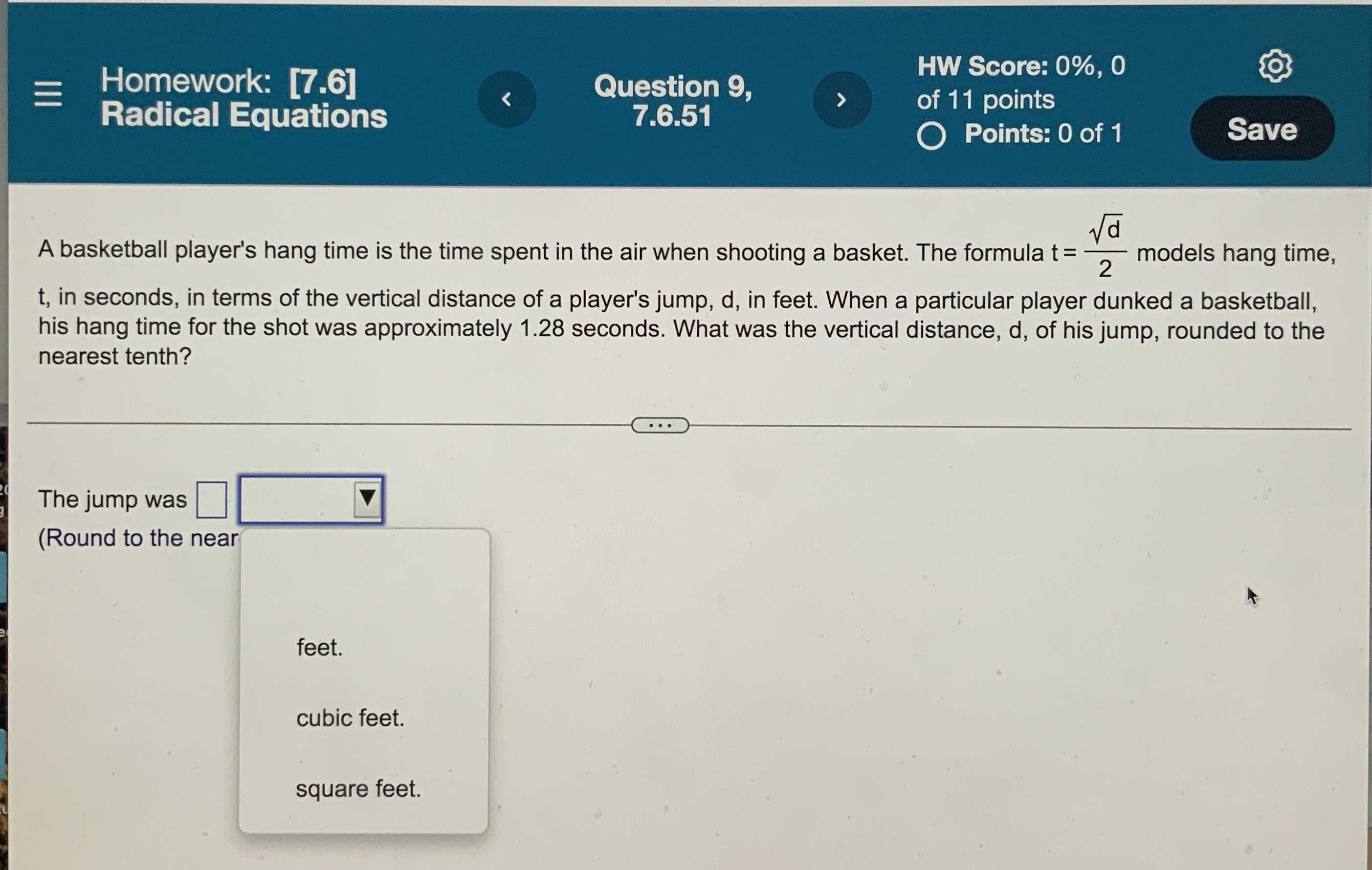### ¿Todavía tienes preguntas de matemáticas?

Pregunte a nuestros tutores expertos
Algebra
PreguntaA basketball player's hang time is the time spent in the air when shooting a basket. The formula $$t = \frac { \sqrt { d } } { 2 }$$ models hang time, $$t$$ , in seconds, in terms of the vertical distance of a player's jump, $$d$$ , in feet. When a particular player dunked a basketball, his hang time for the shot was approximately $$1.28$$ seconds. What was the vertical distance, $$d$$ , of his jump, rounded to the nearest tenth?

The jump was $$\square$$